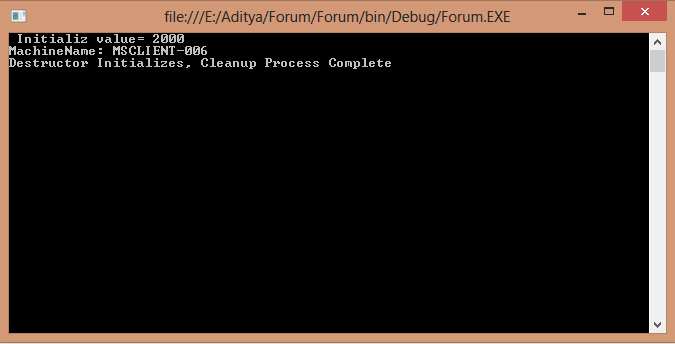# What is Constructor in c#

Posted by  Ankit Singh
1147  View(s)
Ratings:
Rate this:
1.##### Re: What is Constructor in c#

Constructor is a special method of a class which will invoke automatically whenever instance or object of class is created. Constructors are responsible for object initialization and memory allocation of its class. If we create any class without constructor, the compiler will automatically create one default constructor for that class. There is always at least one constructor in every class.

`using System;using System.Collections.Generic;using System.Linq;using System.Text;using System.Threading.Tasks;namespace constructor_initialize{    class Calculate    {        public int temp = 0;        //Constructor Creation        public Calculate(int val)        {            temp = Convert.ToInt32(val);        }        public Calculate(string Message)        {            Console.WriteLine(Message.ToString());        }        public void calculate()        {            decimal Sum = Convert.ToDecimal(temp);            Console.WriteLine(" Initializ value= {0}", temp);        }        //Destructor Initialization, It will clean memory at the end and frees up system resources.        ~Calculate()        {            Console.WriteLine("Destructor Initializes, Cleanup Process Complete");            Console.ReadLine();        }    }    class Program    {        public static void Main(String[] args)        {            Calculate cl = new Calculate(2000);            cl.calculate();            Calculate c2 = new Calculate(string.Format("MachineName: {0}", Environment.MachineName));                  }    }}`Modified On Apr-10-2018 06:17:19 AM